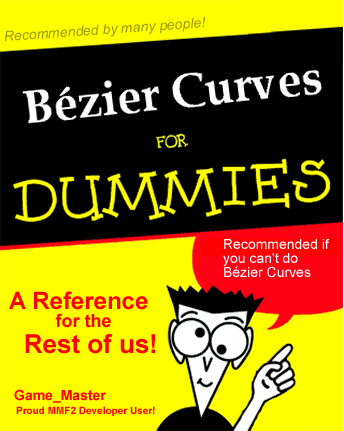# Thread: Bézier Curves for Dummies (TDC version)

1. ## Re: Bézier Curves for Dummies (TDC version)

Nice article, this will help a lot!

I made this because I was bored:2. ## Re: Bézier Curves for Dummies (TDC version)Originally Posted by DanielRehnOriginally Posted by Raylax
One more question, how do I get the moving object to rotate to be facing in the direction it's moving? Preferably using the Set Angle action rather than a 32-direction animationNot that hard really.. If you notice the green line in the following image... it's the angle at the current position of the curveso basically something like this

tx1 = p0x + ((p1x - p0x) * t)
ty1 = p0y + ((p1y - p0y) * t)

tx2 = p1x + ((p2x - p1x) * t)
ty2 = p1y + ((p2y - p1y) * t)

posx = tx1 + ((tx2 - tx1) * t)
posy = ty1 + ((ty2 - ty1) * t)
angle = sqrt( ((tx2 - tx1) pow 2) + ((ty2 - ty1) pow 2) )

I'm a little drunk atm, so I might have gotten a few variables a little wrong x)

Cheers!
Is it the same formula for a Quadratic Bezier?

And, I'm assuming I use the bottom formula and implement the above formulas to replace tx1, tx2 etc. So, it would be:

angle = sqrt( (((p1x + ((p2x - p1x) * t)) - (p0x + ((p1x - p0x) * t))) pow 2) + (((p1y + ((p2y - p1y) * t)
) - (p0y + ((p1y - p0y) * t))) pow 2))

I'm also assuming that p1x, p2x etc etc mean X Position of P1, X Position of P2 and so on.

Am I right on this?

EDIT: And nice cover! :P
I probably qualify under dummy =D3. ## Re: Bézier Curves for Dummies (TDC version)

First of all, as I stated 2 posts below my first one, I accidentally used the distance formula.. replace that with a formula to calc the angle and you should be fine..

also for Quadratic Bezier it's the blue line in the following image...4. ## Re: Bézier Curves for Dummies (TDC version)

A Bezier Curve Extension

So you don't have to remember or implement the formula5. ## Re: Bézier Curves for Dummies (TDC version)

It still does not support angles...6. ## Re: Bézier Curves for Dummies (TDC version)

[size:17pt]New Example[/size]
&nbsp;&nbsp;&nbsp;&nbsp;&nbsp;&nbsp;&nbsp;&nbsp;&n bsp;&nbsp;&nbsp;&nbsp;&nbsp;Multiple Objects&nbsp;&nbsp;&nbsp;&nbsp;&nbsp;&nbsp;&nbsp;& nbsp;&nbsp;&nbsp;&nbsp;&nbsp;&nbsp;&nbsp;&nbsp;&nb sp;&nbsp;&nbsp;&nbsp;&nbsp;&nbsp;&nbsp;&nbsp;&nbsp ;&nbsp;&nbsp;&nbsp;&nbsp;&nbsp;&nbsp;&nbsp;&nbsp;& nbsp;&nbsp;&nbsp;&nbsp;&nbsp;&nbsp;&nbsp;&nbsp;&nb sp;&nbsp;&nbsp;&nbsp;&nbsp;&nbsp;&nbsp;&nbsp;&nbsp ;&nbsp;&nbsp;&nbsp;&nbsp;&nbsp;&nbsp;&nbsp;&nbsp;& nbsp;&nbsp;&nbsp;&nbsp;&nbsp;&nbsp;&nbsp;&nbsp;&nb sp;&nbsp;&nbsp;&nbsp;&nbsp;&nbsp;&nbsp;&nbsp;&nbsp ;&nbsp;&nbsp;&nbsp;&nbsp;&nbsp;&nbsp;&nbsp;&nbsp;& nbsp;&nbsp;&nbsp;&nbsp;&nbsp;&nbsp;&nbsp;&nbsp;&nb sp;&nbsp;&nbsp;&nbsp;

The attached example file shows how to use Object Selection to create many objects, all of whom have a separate Bézier curve.

The example is of three monsters whose heads swivel left to right, on segmented Bézier necks. Assuming you create the same number of neck segments and nodes for each monster, you can have as many copies as you like with no new events.

Note: Advanced. Not recommended for new users, relies heavily on MMF's object selection principles, specifically Many-to-Many relationships within MMF. A prior knowledge in this area is strongly recommended, although a separate frame is provided for you to experiment with the ID relationships in a Many-to-Many environment.

(the long words and jargon are to put the newbies off - trust me guys, if you're new to MMF, this will be beyond you)7. ## Re: Bézier Curves for Dummies (TDC version)

I think the main thing that will scare away new MMF2 users is the expression:

((X( "MBod" )+(X( "MBodDir" )-X( "MBod" ))*t( "MNeck" ))+((X( "MBodDir" )+(X( "MNeckDir" )-X( "MBodDir" ))*t( "MNeck" ))-(X( "MBod" )+(X( "MBodDir" )-X( "MBod" ))*t( "MNeck" )))*t( "MNeck" ))+(((X( "MBodDir" )+(X( "MNeckDir" )-X( "MBodDir" ))*t( "MNeck" ))+((X( "MNeckDir" )+(X( "MHead" )-X( "MNeckDir" ))*t( "MNeck" ))-(X( "MBodDir" )+(X( "MNeckDir" )-X( "MBodDir" ))*t( "MNeck" )))*t( "MNeck" ))-((X( "MBod" )+(X( "MBodDir" )-X( "MBod" ))*t( "MNeck" ))+((X( "MBodDir" )+(X( "MNeckDir" )-X( "MBodDir" ))*t( "MNeck" ))-(X( "MBod" )+(X( "MBodDir" )-X( "MBod" ))*t( "MNeck" )))*t( "MNeck" )))*t( "MNeck" )

While it is fairly simple in principle and concept, it can be intimidating if judged on looks alone.8. ## Re: Bézier Curves for Dummies (TDC version)

Yeah, it's worse since I used E++ to write that, and I think E++ is generating pretty unoptimised expressions right now, lolSo it probably needs half as many brackets.####Posting Permissions

• You may not post new threads
• You may not post replies
• You may not post attachments
• You may not edit your posts
•